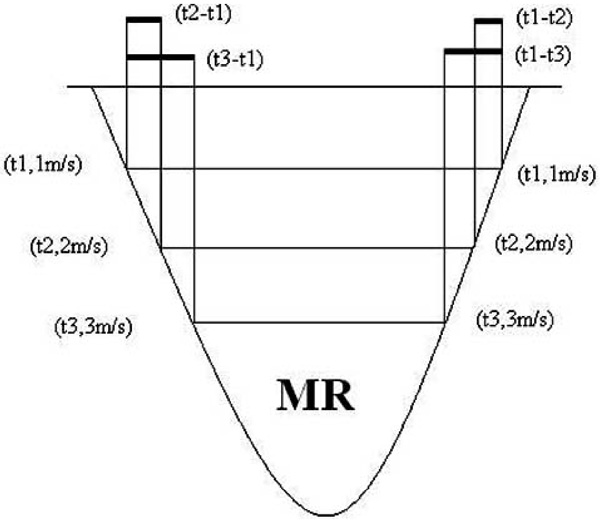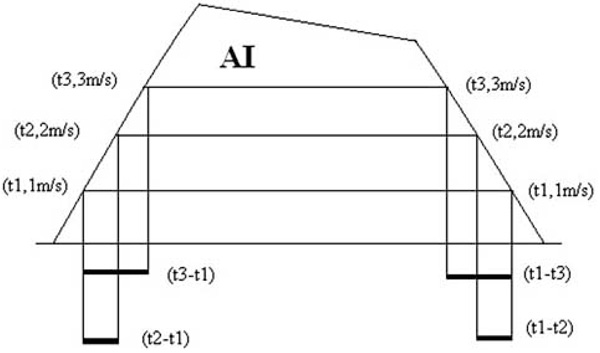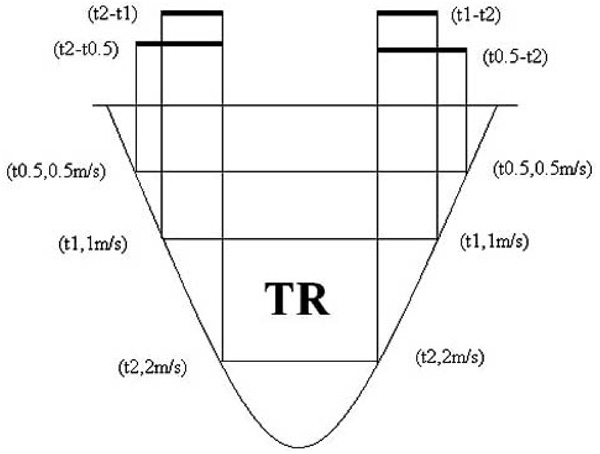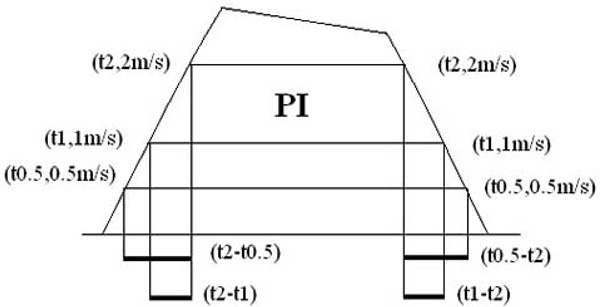# Time Constants of Cardiac Function and Their Calculations

Xufang Bai1, *, Quan Wang2
1 University of Ottawa Heart Institute, Ottawa, ON, Canada
2 Quan Wang, Department of Mechanical and Manufacturing Engineering, University of Manitoba, Winnipeg, MB, Canada

#### Article Metrics

9
##### Total Statistics:

Full-Text HTML Views: 1355
Abstract HTML Views: 546
##### Unique Statistics:

Full-Text HTML Views: 683
Abstract HTML Views: 327open-access license: This is an open access article licensed under the terms of the Creative Commons Attribution Non-Commercial License (http://creativecommons.org/licenses/by-nc/3.0/) which permits unrestricted, non-commercial use, distribution and reproduction in any medium, provided the work is properly cited.

* Address correspondence to this author at the University of Ottawa Heart Institute, 40 Ruskin Street, Ottawa, Ontario, K1Y 4W7, Canada; Tel: 613-761-5000; Fax: 613-761-4695; E-mail: xufangbai@yahoo.com

## Abstract

Left ventricular diastolic time constant, Tau, is the most established index to describe left ventricular diastolic function. However, the lack of a practical method for the measurement of Tau has been an uncomfortable reality which formerly kept all but a few researchers from making use of it. Recently, the non invasive calculation of Tau in an echo lab was accomplished through formulas developed by universal mathematical method. Tau was first suggested by the fact that left ventricular diastole is an active process, and we can therefore predict that there must be some other time constants which can be used to describe other active movement of ventricular muscles during isovolumic period. Similar mathematical manipulation was employed to develop formulas for “the other Tau(s)”. Such Tau(s) represent new sets of indexes useful for the description of cardiac function. They are expected to be the most established indices given the fact Tau is revealing the power of ventricular muscles without interference from either preload or afterload.

Keywords: Tau, cardiac function, left ventricular diastolic time constant..

## INTRODUCTION

In 1976, Weiss et al.  found that left ventricular diastolic function can be described by an index called left ventricular diastolic time constant, or Tau, which is given by

(1)  $p={e}^{-t/T+B}$

Where P is the pressure within the left ventricle; e is the base of natural logarithm, e=2.718281828…; t is the time from –dp/dt max, shortly after aortic valves closure; T is the left ventricular diastolic time constant, or Tau; B is a constant. From Eq. (1), the derivative of both sides is expressed by

(2a)  $\mathrm{dP}=\left({e}^{-t/T+B}\right)\left(-t/T\right)\mathrm{dt}=P\left(-1/T\right)\mathrm{dt}$

Or:

(2b)  $T=-P/\left(\mathrm{dp}/\mathrm{dt}\right)$

Since both P and dp/dt are variants which can be conveniently measured from catheter labs, Tau can be calculated invasively there. However clinicians in catheter labs are more interested in treatment rather than diagnosis. Since 1992, some endeavors have been attempted for non-invasive measurement of Tau in leading echo labs, [2-5] which led to the equation recommended by ASE 2009 diastolic function guideline: 

(3)

Where IVRT is isovolumic relaxation time. While everyone agrees that Tau is the most established index to describe left ventricular diastolic function, [2-5] few clinicians use it in daily clinical practice. In addition, the deduction process of this equation is based on many assumptions.  Another issue needing to be addressed is Weiss’ formula which is based on the zero asymptote model. It is commonly believed that the non-zero asymptote model is better while the zero asymptote model is merely acceptable.  In non-zero asymptote model, Weiss’ formula was modified:

(4)  $p={e}^{-t/T+B}+C$

Where C is another constant, the asymptote.

Recently, based on Weiss’ formula, the simplified Bernoulli equation and universal mathematical methods, new easy-to-handle formulas were developed to calculate Tau. [9, 10] Furthermore, formulas can be developed even with the non-zero asymptote model. The method is briefly introduced below:

### 1. In Mitral Regurgitation Patients:

In the Echo examination for patients with mitral regurgitation, the left ventricular pressure can be expressed as:

(5LDM)  $p=P+\mathrm{LAP}$

Where ΔP is the pressure gradient between left atrium and left ventricle; LDM is for left ventricular diastolic time constant in mitral regurgitation patients. Substituting Eq. (4) into Eq. (5LDM) and employing simplified Bernoulli’s equation:

ΔP=4v2 leads to the following equation:

e-t/T+B+ C = ΔP + LAP = 4v2 + LAP

Or:

(6LDM)  ${e}^{tT+B}=4{v}^{2}+\mathrm{LAP}C$

A natural logarithmic transformation on both sides of the above equation results in the expression:

(7LDM)  $tT+B=1n\left(4{v}^{2}+\mathrm{LAP}C\right)$

Three points, (t1, 1m/s), (t2, 2m/s) and (t3, 3m/s), are then chosen on the descending limb of the mitral regurgitation continuous-wave Doppler velocity curve Fig. (1), which produces the following three equations:Fig. (1). A depiction of continuous wave Doppler mitral regurgitation spectrum where left ventricular diastolic time constant is calculated on the descending branch (right); left ventricular systolic time constant is calculated on the ascending branch (left).Fig. (2). A depiction of continuous wave Doppler aortic regurgitation (insufficiency) spectrum where left ventricular diastolic time constant is calculated on the ascending branch (left); left ventricular systolic time constant is calculated on the descending branch (right).Fig. (3). A depiction of continuous wave Doppler tricuspid regurgitation spectrum where right ventricular diastolic time constant is calculated on the descending branch (right); right ventricular systolic time constant is calculated on the ascending branch (left).Fig. (4). A depiction of continuous wave Doppler pulmonary regurgitation (insufficiency) spectrum where right ventricular diastolic time constant is calculated on the ascending branch (left); right ventricular systolic time constant is calculated on the descending branch (right).

(8LDM1)  $t1/TB=1n\left(4{1}^{2}\mathrm{LAP}C\right)$

(8LDM2)  $t2/TB=1n\left(4{2}^{2}\mathrm{LAP}C\right)$

(8LDM3)  $t3/TB=1n\left(4{3}^{2}\mathrm{LAP}C\right)$

From the difference comparison of Eqs. (8LDM1) and (8LDM2), we find:

$\left(t1t2\right)T=1n\left(4{1}^{2}+\mathrm{LAP}C\right)1n\left(4{2}^{2}+\mathrm{LAP}C\right)$

Or:

(9LDM1)  $\mathrm{Tau}=\left(t1t2\right)1n\left(\left(16+\mathrm{LAP}C\right)\left(4+\mathrm{LAP}C\right)\right)$

Similarly,

(9LDM2)  $\mathrm{Tau}=\left(t1t3\right)1n\left(\left(36+\mathrm{LAP}C\right)\left(4+\mathrm{LAP}C\right)\right)$

From the above formulas (9LDM1) and (9LDM2), both Tau and (LAP-C) can be solved after we measure two time intervals: (t1-t3) and (t1-t2). Fig. (1) shows how measurement is done.

### 2. In Aortic Regurgitation Patients:

In the Echo examination for patients with aortic regurgitation, the left ventricular pressure can be expressed as:

(5LDA)  $P=\mathrm{ADP}P$

Where ADP is the aortic diastolic pressure, ΔP is the pressure gradient between aorta and left ventricle; LDA is for left ventricular diastolic time constant in aortic regurgitation patients. Substituting Eq. (4) into Eq. (5LDA) and employing the simplified Bernoulli’s equation: ΔP=4×v2 leads to the following equation:

${e}^{tT+B}+C=\mathrm{ADP}P=\mathrm{ADP}4{v}^{2}$

Or:

(6LDA)  ${e}^{t/TB}=\mathrm{ADP}4{v}^{2}C$

A natural logarithmic transformation on both sides of the above equation results in the expression:

(7LDA)  $tT+B=1n\left(\mathrm{ADP}4{v}^{2}C\right)$

Three points, (t1, 1m/s), (t2, 2m/s) and (t3, 3m/s), are chosen on the ascending limb of the aortic regurgitation continuous-wave Doppler velocity curve Fig. (2), and substituted into Eq. (7LDA) respectively, which produces the following three equations:

(8LDA1)  $t1T+B=1n\left(\mathrm{ADP}4{1}^{2}C\right)$

(8LDA2)  $\mathrm{t2}TB=1n\left(\mathrm{ADP}4{2}^{2}C\right)$

(8LDA3)  $\mathrm{t3}TB=1n\left(\mathrm{ADP}4{3}^{2}C\right)$

From the difference comparison of Eqs. (8LDA1) and (8LDA2), we find:

$\left(t1t2\right)T=1n\left(\mathrm{ADP}4{1}^{2}C\right)ln\left(\mathrm{ADP}4{2}^{2}C\right)$

Or:

(9LDA1)  $\mathrm{Tau}=\left(t2t1\right)1n\left(\left(\mathrm{ADP}C4\right)\left(\mathrm{ADP}C16\right)\right)$

Similarly,

(9LDA2)  $\mathrm{Tau}=\left(t3t1\right)/1n\left(\left(\mathrm{ADP}C4\right)/\left(\mathrm{ADP}C36\right)\right)$

From the above formulas (9LDA1) and (9LDA2), both Tau and (ADP-C) can be solved after we measure two time intervals: (t3-t1) and (t2-t1). Fig. (2) shows how measurement is done.

In terms of location and time, cardiac function includes right ventricular diastolic function, right ventricular systolic function, left ventricular diastolic function and left ventricular systolic function. Each of the above mentioned “cardiac functions” begins with an isovolumic period when both the inflow and outflow valves close simultaneously. Apparently, this isovolumic period is a golden period to measure cardiac function since ventricular pressure change is not influenced by either preload or afterload.

Our hypothesis is that the time constant can be extended to all other “cardiac functions”. This means Eq. (4) is not limited to left ventricular diastole, but can also be applied in left ventricular systole, right ventricular diastole and right ventricular systole. Correspondingly, we will have the left ventricular systolic time constant, right ventricular diastolic time constant and right ventricular systolic time constant.

Such a hypothesis is based on the following premises: 1. Left ventricular diastolic time constant is suggested because left ventricular diastolic process is an active process.  Left ventricular systolic process is also an active process, so are right ventricular diastolic and systolic processes. 2. During isovolumic period, the ventricle is like a sealed box with both inflow and outflow valves shut off. With the active ventricular muscle movement, it is sensible to imagine the pressure built within it is described by an index equation. 3. In continuous wave Doppler regurgitation spectra, all of the paired ascending and descending branches are almost symmetric, showing they experience a similar process and can be characterized by a time constant which dictates how “fast” the pressure changes within the ventricle.

Attempts are being made to use a similar strategy to develop new formulas to calculate the time constants for the other “cardiac functions”.

## DEDUCTION OF FORMULAS FOR OTHER TIME CONSTANTS

### 1. Left Ventricular Systolic Time Constant

• In mitral regurgitation patients
Formulas for the calculation of left ventricular systolic time constant in mitral regurgitation patients are developed with a similar mathematical method, except:
Three points, (t1, 1m/s), (t2, 2m/s) and (t3, 3m/s), are chosen on the ascending limb of the mitral regurgitation continuous-wave Doppler velocity curve Fig. (1). The final formulas are:

(9LSM1)  $\mathrm{Tau}=\left(t2t1\right)1n\left(\left(4+\mathrm{LAP}C\right)\left(16+\mathrm{LAP}C\right)\right)$

(9LSM2)  $\mathrm{Tau}=\left(t3t1\right)1n\left(\left(4+\mathrm{LAP}C\right)\left(36+\mathrm{LAP}C\right)\right)$

where LSM is for left ventricular systolic time constant in mitral regurgitation patients.
• In aortic regurgitation patients
Formulas for the calculation of left ventricular systolic time constant in aortic regurgitation patients are developed with a similar mathematical method, except:
Three points, (t1, 1m/s), (t2, 2m/s) and (t3, 3m/s), are chosen on the descending limb of the aortic regurgitation continuous-wave Doppler velocity curve Fig. (2). The final formulas are:

(9LSA1)  $\mathrm{Tau}=\left(t1t2\right)/1n\left(\left(\mathrm{ADP}C16\right)/\left(\mathrm{ADP}C4\right)\right)$

(9LSA2)  $\mathrm{Tau}=\left(t1t3\right)/1n\left(\left(\mathrm{ADP}C36\right)/\left(\mathrm{ADP}C4\right)\right)$

Where LSA is for left ventricular systolic time constant in aortic regurgitation patients.

### 2. Right Ventricular Diastolic Time Constant

• In tricuspid regurgitation patients
Formulas for the calculation of right ventricular diastolic time constant in tricuspid regurgitation patients are developed with a similar mathematical method, except:
Three points, (t0.5, 0.5m/s), (t1, 1m/s) and (t2, 2m/s), are chosen on the descending limb of the tricuspid regurgitation continuous-wave Doppler velocity curve Fig. (3). The final formulas are:

(9RDT1)  $\mathrm{Tau}=\left(t0.5t2\right)/1n\left(\left(16+\mathrm{RAP}C\right)/\left(1+\mathrm{RAP}C\right)\right)$

(9RDT2)  $\mathrm{Tau}=\left(t1t2\right)/1n\left(\left(16+\mathrm{RAP}C\right)/\left(4+\mathrm{RAP}C\right)\right)$

Where RAP is for right atrial pressure and RDT is right ventricular diastolic time constant in tricuspid regurgitation patients.
• In pulmonary regurgitation patients
Formulas for the calculation of right ventricular diastolic time constant in pulmonary regurgitation patients are developed with a similar mathematical method, except:
Three points, (t0.5, 0.5m/s), (t1, 1m/s) and (t2, 2m/s), are chosen on the ascending limb of the pulmonary regurgitation continuous-wave Doppler velocity curve Fig. (4). The final formulas are:

(9RDP)  $\mathrm{Tau}=\left(t2t1\right)/1n\left(\left(\mathrm{PDP}C4\right)/\left(\mathrm{PDP}C16\right)\right)$

(9RDP)  $\mathrm{Tau}=\left(t2t0.5\right)/1n\left(\left(\mathrm{PDP}C1\right)/\left(\mathrm{PDP}C16\right)\right)$

Where PDP is for pulmonary diastolic pressure and RDP is right ventricular diastolic time constant in pulmonary regurgitation patients.

### 3. Right Ventricular Systolic Time Constant

• In tricuspid regurgitation patients
Formulas for the calculation of right ventricular systolic time constant in tricuspid regurgitation patients are developed with a similar mathematical method, except:
Three points, (t0.5, 0.5m/s), (t1, 1m/s) and (t2, 2m/s), are chosen on the ascending limb of the tricuspid regurgitation continuous-wave Doppler velocity curve Fig. (3). The final formulas are:

(9RST1)  $\mathrm{Tau}=\left(t2t1\right)/1n\left(\left(4+\mathrm{RAP}C\right)/\left(16+\mathrm{RAP}C\right)\right)$

(9RST2)  $\mathrm{Tau}=\left(t2t0.5\right)/1n\left(\left(1+\mathrm{RAP}C\right)/\left(16+\mathrm{RAP}C\right)\right)$

where RST is for right ventricular systolic time constant in tricuspid regurgitation patients.
• In pulmonary regurgitation patients
Formulas for the calculation of right ventricular systolic time constant in pulmonary regurgitation patients are developed with a similar mathematical method, except:
Three points, (t0.5, 0.5m/s), (t1, 1m/s) and (t2, 2m/s), are chosen on the descending limb of the pulmonary regurgitation continuous-wave Doppler velocity curve Fig. (4). The final formulas are:

(9RSP1)  $\mathrm{Tau}=\left(t1t2\right)/1n\left(\left(\mathrm{PDP}C16\right)/\left(\mathrm{PDP}C4\right)\right)$

(9RSP2)  $\mathrm{Tau}=\left(t0.5t2\right)/1n\left(\left(\mathrm{PDP}C16\right)/\left(\mathrm{PDP}C1\right)\right)$

where RSP is for right ventricular systolic time constant in pulmonary regurgitation patients.

## “DP/DT” SHOULD BE REPLACED BY MEASUREMENT OF TAU

Measurement of dp/dt is very popular at this time. [11, 12] It is more reasonable than measurement of mean dp/dt, the ratio of the change in pressure during isovolumic contraction/dilation over the isovolumic time. However, there is still a big gap between the “dp/dt” and dp/dt max, which is a well accepted index, which, until now, can not be measured conveniently. Actually, dp/dt is a one point value, which means there are infinite dp/dt along the curve and each one is unique. In terms of the description of cardiac function, dp/dt max is equivalent to Tau. To show this, let us compare left ventricular diastolic time constant and –dp/dt max by taking a look of Eq. (4) again:

(4)  $P={e}^{tT+B}+C$

The derivative of both sides is expressed by:

$\mathrm{dp}={e}^{tT+B}\left(1T\right)\mathrm{dt}$

Since t starts from dp/dt max,  when t=0, we have:

Because eB is a constant, we can draw the conclusion that dp/dt max and Tau are equivalent indices. That also means measurement of left ventricular systolic time constant is better than measurement of dp/dt of left ventricular contractility; measurement of right ventricular systolic time constant is better than measurement of dp/dt of right ventricular contractility.

## SOME CONSIDERATIONS COMING WITH APPLICATION

1. All the above formulas can be integrated into Echo machines, such that Tau can be calculated automatically after two time intervals have been measured on the ascending or descending branch of regurgitation spectrum.
2. Quick scan speed, i.e. 200mm/s is strongly recommended to ensure that time intervals are measured accurately. Pedoff probe is a good choice for the same reason. Accurate measurement is the key. We predict with the help of computer technology accurate measurement instead of our daily manual measurement will give us more surprises in both basic research and clinical practice of echocardiography.
3. No ventricular pressure change caused by regurgitation is expected when we develop these formulas, which means severe regurgitation contributing extra pressure change might unexpectedly cause compromised results. Conversely, too mild regurgitation will not give clear ascending or descending branches for reliable measurement of time intervals. The minimum regurgitation volume rendering satisfactory measurement constructs the optimizing scenario. Sonic contrast is helpful to enhance the silhouette of interests.
4. Right heart regurgitation is far more common than left heart regurgitation. That means we have more chances to inspect right heart function in the Tau point of view.
5. Except left ventricular diastolic time constant, the normal values of other time constants and their relevant clinical involvement are all unknowns so far. More research is required to further understand cardiac function and how it compromises under pathological circumstances. Since the left ventricular diastolic time constant is the most established index to describe left ventricular diastolic function, we believe all the other time constants will be the best indexes to describe other cardiac functions.
6. Simultaneous catheter verification study is encouraged to allow these formulas to receive extensive mainstream acceptance.

## ACKNOWLEDGEMENT

Thanks to Seth Wynes for the preparation of this manuscript.

## REFERENCES

  Weiss JL, Frederiksen JW, Weisfeldt ML. Hemodynamic determinants of the time-course of fall in canine left ventricular pressure J Clin Invest 1976; 58: 751-60.  Chen C, Rodriguez L, Levine RA, Weyman AE, Thomas JD. Noninvasive measurement of the time constant of left ventricular relaxation using the continuous-wave Doppler velocity profile of mitral regurgitation Circulation 1992; 86: 272-8.  Nishimura RA, Schwartz RS, Tajik AJ, Holmes DR Jr. Noninvasive measurement of rate of left ventricular relaxation by Doppler Echocardiography. Validation with simultaneous cardiac catheterization Circulation 1993; 88: 146-55.  Thomas JD, Flachskampf FA, Chen C, et al. Isovolumic relaxation time varies predictably with its time constant and aortic and left atrial pressure: implications for the noninvasive evaluation of ventricular relaxation Am Heart J 1992; 124: 1305-.  Scalia GM, Greenberg NL, McCarthy PM, Thomas JD, Vandervoort PM. Noninvasive assessment of the ventricular relaxation time constant in humans by Doppler Echocardiography Circulation 1997; 95: 151-5.  Nagueh SF, Appleton CP, Gillebert TC, et al. Recommendations for the evaluation of left ventricular diastolic function by echocardiography JASE 2009; 22: 107-33.  Bai X. Are we on the right way to calculate Tau? JASE 2009; 22: 859.  Langer SFJ, Habazettl H. Estimation of the left ventricular relaxation time constant Tau requires consideration of the pressure asymptote Physiol Res 2005; 54: 601-10.  Bai X. Calculation of left ventricular relaxation time constant-Tau in patients with mitral regurgitation by continuous-wave Doppler Open Cardiovasc Med J 2008; 2: 9-11.  Bai X. Calculation of Left Ventricular Relaxation Time Constant-Tau in Patients With Aortic Regurgitation by Continuous-Wave Doppler Open Cardiovasc Med J 2008; 2: 28-30.  Bargiggia GS, Bertucci C, Recusani F, et al. A new method for estimating left ventricular dP/dt by continuous wave Doppler-echocardiography. Validation studies at cardiac catheterization Circulation 1989; 80: 1287-92.  Oh JK, Seward JB, Tajik AJ, Eds. The Echo Manual. 2nd. Lippincott: Williams & Wilkins 1999.